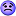# Another Integral Challenge

• I

## Main Question or Discussion Point

I'm up against this Laplace transform integral:
$$F(s) ~:=~ \int^\infty_0 \exp\left( -sx + \frac{i\omega}{1+\lambda x} \right) \, dx$$where $s$ is complex, $\omega$ is a real constant, and $\lambda$ is a positive real constant.

By inspection, I think it should converge, at least for some (nontrivial) domain of values for $s$ and $\omega$ (tell me if I'm wrong). But every symbolic integrator I've tried barfs on it.

I figured I should at least ask here before I give up.mathman
The only condition you need is the real part of s is > 0.

Even so, I still don't know how to perform the integral (other than numerically -- but I want a closed form symbolic expression).